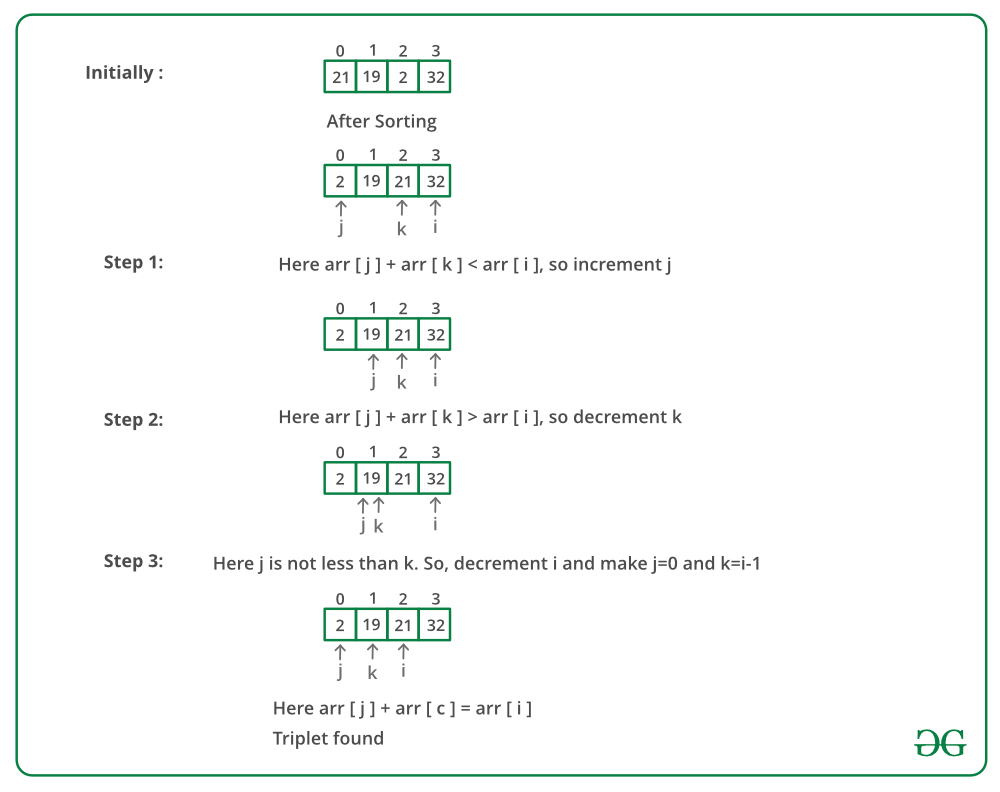# Javascript Program to Find a triplet such that sum of two equals to third element

Given an array of integers, you have to find three numbers such that the sum of two elements equals the third element.
Examples:

```Input: {5, 32, 1, 7, 10, 50, 19, 21, 2}
Output: 21, 2, 19

Input: {5, 32, 1, 7, 10, 50, 19, 21, 0}
Output: no such triplet exist```

Question source: Arcesium Interview Experience | Set 7 (On campus for Internship)

Simple approach: Run three loops and check if there exists a triplet such that sum of two elements equals the third element.
Time complexity: O(n^3)
Efficient approach: The idea is similar to Find a triplet that sum to a given value.

• Sort the given array first.
• Start fixing the greatest element of three from the back and traverse the array to find the other two numbers which sum up to the third element.
• Take two pointers j(from front) and k(initially i-1) to find the smallest of the two number and from i-1 to find the largest of the two remaining numbers
• If the addition of both the numbers is still less than A[i], then we need to increase the value of the summation of two numbers, thereby increasing the j pointer, so as to increase the value of A[j] + A[k].
• If the addition of both the numbers is more than A[i], then we need to decrease the value of the summation of two numbers, thereby decrease the k pointer so as to decrease the overall value of A[j] + A[k].

Below image is a dry run of the above approach:Below is the implementation of the above approach:

## Javascript

 ``

Output:

`numbers are 21 2 19`

Time complexity: O(N^2)

Space Complexity: O(1) as no extra space has been used.

Another Approach: The idea is similar to previous approach.

1. Sort the given array.
2. Start a nested loop, fixing the first element i(from 0 to n-1) and moving the other one j (from i+1 to n-1).
3. Take the sum of both the elements and search it in the remaining array using Binary Search.

## Javascript

 `` `// This code is contributed by Sarthak Delori`

Time Complexity: O(N^2*log N)

Space Complexity:  O(1)

Please refer complete article on Find a triplet such that sum of two equals to third element for more details!

Feeling lost in the world of random DSA topics, wasting time without progress? It's time for a change! Join our DSA course, where we'll guide you on an exciting journey to master DSA efficiently and on schedule.
Ready to dive in? Explore our Free Demo Content and join our DSA course, trusted by over 100,000 geeks!

Previous
Next